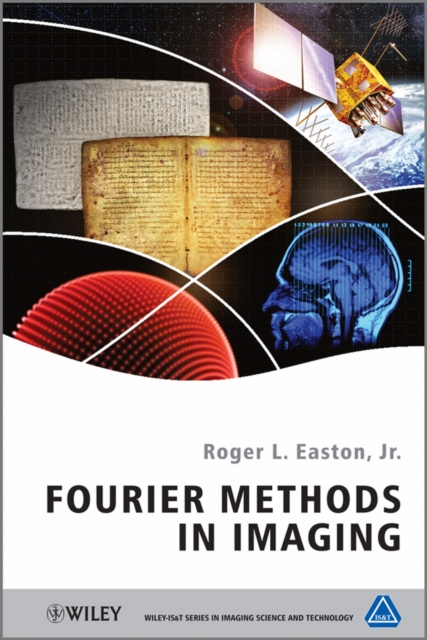# Fourier Methods in Imaging Hardback

## Part of the The Wiley-IS&T Series in Imaging Science and Technology series

Hardback

• Information

#### Description

Fourier Methods in Imaging introduces the mathematical tools for modeling linear imaging systems to predict the action of the system or for solving for the input.

The chapters are grouped into five sections, the first introduces the imaging tasks (direct, inverse, and system analysis), the basic concepts of linear algebra for vectors and functions, including complex-valued vectors, and inner products of vectors and functions.

The second section defines "special" functions, mathematical operations, and transformations that are useful for describing imaging systems.

Among these are the Fourier transforms of 1-D and 2-D function, and the Hankel and Radon transforms.

This section also considers approximations of the Fourier transform.

The third and fourth sections examine the discrete Fourier transform and the description of imaging systems as linear "filters", including the inverse, matched, Wiener and Wiener-Helstrom filters.

The final section examines applications of linear system models to optical imaging systems, including holography. * Provides a unified mathematical description of imaging systems. * Develops a consistent mathematical formalism for characterizing imaging systems. * Helps the reader develop an intuitive grasp of the most common mathematical methods, useful for describing the action of general linear systems on signals of one or more spatial dimensions. * Offers parallel descriptions of continuous and discrete cases. * Includes many graphical and pictorial examples to illustrate the concepts.

This book helps students develop an understanding of mathematical tools for describing general one- and two-dimensional linear imaging systems, and will also serve as a reference for engineers and scientists

£121.75

£93.45

£83.75

£65.64

£118.50

£91.06

£94.50

£73.51

£90.50

£70.58